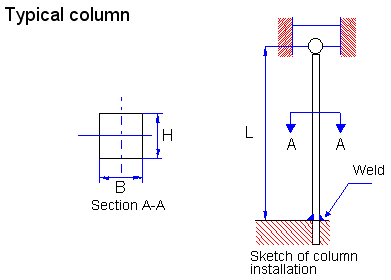## Posts

Showing posts from October, 2007

### Improve math skills of your kids - Learn step-by-step arithmetic from Math games

Math: Unknown - Step-by-step math calculation game for iOS.

Math: Unknown is much more than a math game. It is a step-by-step math calculation game which will teach users how to calculate in the correct order rather than just asking only the final calculated results.

The app consists of four basic arithmetic operations which are addition, subtraction, multiplication and division. In order to get started, users who are new to arithmetic can learn from animated calculation guides showing step-by-step procedures of solving each type of operation. It is also helpful for experienced users as a quick reference.

Generally, addition and subtraction may be difficult for users who just start learning math especially when questions require carrying or borrowing (also called regrouping). The app helps users to visualize the process of carrying and borrowing in the way it will be done on paper. Once users understand how these operations work, they are ready to learn multiplication and division.

For most students, division is considered as the most difficult arithmetic operation to solve. It is a common area of struggle since it requires prior knowledge of both multiplication and subtraction. To help users understand division, the app uses long division to teach all calculation procedures. Relevant multiplication table will be shown beside the question. Users will have to pick a number from the table which go into the dividend. Multiplication of selected number and divisor is automatically calculated, but the users have to do subtraction and drop down the next digit themselves. Learning whole calculation processes will make them master it in no time.

Math: Unknown is a helpful app for students who seriously want to improve arithmetic calculation skills.

### Column design and analysisIn a design situation, the expected load on a column would be known, along with the length required by the application. The designer would then specify the following:
1. The manner of attaching the ends to the structure that affects the end fixity.
2. The general shape of the column cross section (for example, round, square, rectangular, and hollow tube).
3. The material for the column.
4. The design factor, considering the application.
5. The final dimensions for the column.
It may be desirable to propose and analyze several different designs to approach an optimum for the application, so software such as this facilitates the process.
It is assumed that the designer for any given trial specifies items 1 through 4. For some simple shapes, such as the solid round or square section, the final dimensions are computed from the appropriate formula: the Euler formula, or the J. B. Johnson formula. If an algebraic solution is not possible, iteration can be done.
In a design situation, the u…

### Stresses and Deformations in BeamsReactions are the forces and/or couples acting at the supports and holding the beam and holding the beam in place. In some cases the user should enter a distributed load to account for the weight of the beam.

The shear V effective on a section is the algebraic sum of all forces acting parallel to and on one side of the section,

The bending moment is the algebraic sum of the moments due to applied loads and other applied moments to one side of the section of interest. Using value V bending moment can be calculated where x = position on the beam measured along its length
M0 = constant of integration evaluated from the boundary conditions.
A bending moment that bends a beam convex downward (tensile stress on bottom fiber) is considered positive, while convex upward (compressive on bottom fiber) is negative.
Moment and shear diagram constructed by plotting to scale the particular entity as the ordinate for each section of the beam. Such diagrams show in continuous form the variation among the …

### FastenersA set of n bolts is to be used to provide a clamping force of F between two components. The load is shared equally among the bolts. Specify suitable bolts, including the grade of the material, if each is to be stressed to K % of its proof strength. The variable K is called the demand factor.

The load on each screw is to be
P = F /n

Specify a bolt made from an SAE grade steel, having a proof strength s psi. Then the allowable stress is
sa = K.[s]

The required tensile stress area for the bolt is then
At = P / sa

From a table find the required tensile stress area for the thread. The required tightening torque will be
T = k1 x D x P

where
D - nominal outside diameter of threads